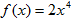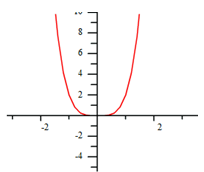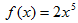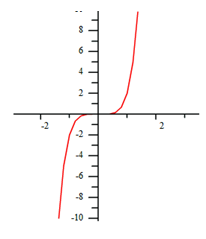Functions Where p > 1

When p > 1 and an even number, the shape of the graph will be like a parabola. If the k-value is positive the graph will open up and y will approach positive infinity as x approaches both positive and negative infinity. If the k-value is negative the graph will open down and y will approach negative infinity as x approaches both positive and negative infinity. The graph below represents the function,.When p > 1 and an odd number, the shape of the graph will be like a cubic function. If the k-value is positive, the graph will be increasing from left to right, as x approaches positive infinity so will y, and vice versa. If the k-value is negative the graph will be decreasing from left to right, as x approaches positive infinity, y will approach negative infinity, and vice versa. The graph below represents the function,.Listen